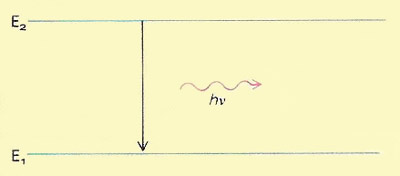A# quantumA quantum is a discrete quantity of energy associated with a wave of frequency ν, where h is Planck's constant. It is the smallest amount of energy that can be absorbed or radiated by matter at that frequency.

Fundamental to quantum theory is the notion that energy is quantized: that material systems can exist only in certain discrete and defined energy states and that transitions between these states can only occur by the gain or loss or particular quantities of energy. In the accompanying diagram a system in energy state E2 changes to energy state E1, emitting electromagnetic radiation in the process. The frequency (ν) of this radiation is determined by the energy change (E2 - E1) involved in the transition according to the equation E2 - E1 = .